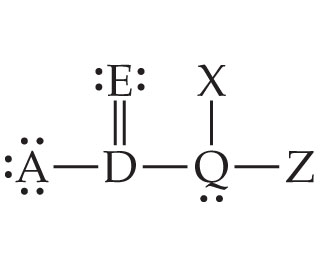# Problem: In the Lewis structure shown here, A, D, E, Q, X, and Z represent elements in the first two rows of the periodic table (H - Ne).Identify Z so that its formal charge is zero.

###### FREE Expert Solution
99% (354 ratings)
###### Problem Details
In the Lewis structure shown here, A, D, E, Q, X, and Z represent elements in the first two rows of the periodic table (H - Ne).Identify Z so that its formal charge is zero.

Frequently Asked Questions

What scientific concept do you need to know in order to solve this problem?

Our tutors have indicated that to solve this problem you will need to apply the Formal Charge concept. You can view video lessons to learn Formal Charge. Or if you need more Formal Charge practice, you can also practice Formal Charge practice problems.